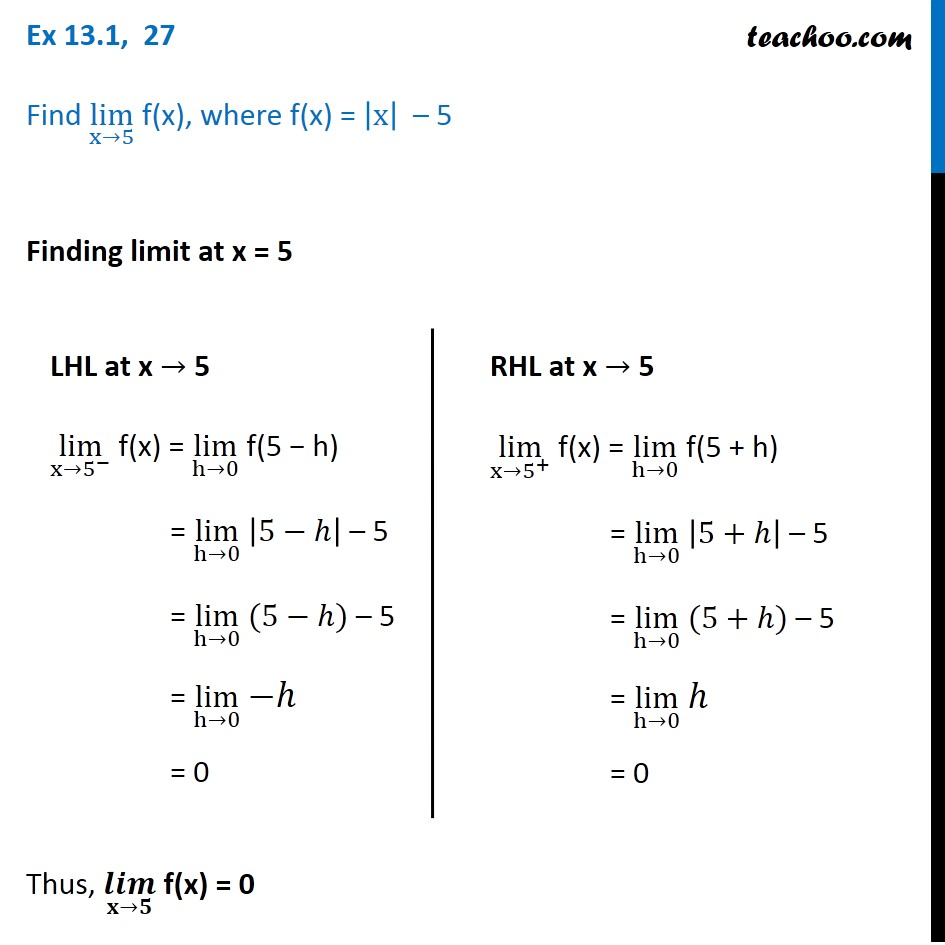1. Chapter 13 Class 11 Limits and Derivatives (Term 1 and Term 2)
2. Serial order wise
3. Ex 13.1 (Term 1)

Transcript

Ex 13.1, 27 Find lim┬(x→5) f(x), where f(x) = |x| – 5 Finding limit at x = 5 LHL at x → 5 lim┬(x→5^− ) f(x) = lim┬(h→0) f(5 − h) = lim┬(h→0) |5−ℎ| – 5 = lim┬(h→0) (5−ℎ) – 5 = lim┬(h→0) −ℎ = 0 RHL at x → 5 lim┬(x→5^+ ) f(x) = lim┬(h→0) f(5 + h) = lim┬(h→0) |5+ℎ| – 5 = lim┬(h→0) (5+ℎ) – 5 = lim┬(h→0) ℎ = 0 Thus, (𝒍𝒊𝒎)┬(𝐱→𝟓) f(x) = 0